## Chinese Remainder Theorem

Letandbe Positive Integers which are Relatively Prime and letandbe any two Integers. Then there is an Integer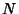such that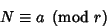(1)

and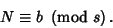(2)

Moreover,is uniquely determined modulo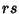. An equivalent statement is that if, then every pair of Residue Classes moduloandcorresponds to a simple Residue Class modulo.

The theorem can also be generalized as follows. Given a set of simultaneous Congruences(3)

for, ...,and for which theare pairwise Relatively Prime, the solution of the set of Congruences is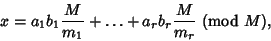(4)

where(5)

and theare determined from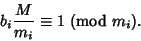(6)

References

Ireland, K. and Rosen, M. The Chinese Remainder Theorem.'' §3.4 in A Classical Introduction to Modern Number Theory, 2nd ed. New York: Springer-Verlag, pp. 34-38, 1990.

Uspensky, J. V. and Heaslet, M. A. Elementary Number Theory. New York: McGraw-Hill, pp. 189-191, 1939.

Wagon, S. The Chinese Remainder Theorem.'' §8.4 in Mathematica in Action. New York: W. H. Freeman, pp. 260-263, 1991.